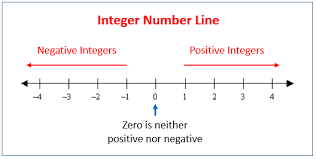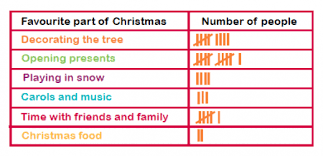We have consolidated the syllabus for Class 7 Maths, for a learner’s quicker and better understanding.

## Chapter 1 – Integers

Integers are a collection of numbers that consist of all the natural numbers (0, 1, 2, 3, 4, and so on) and their negatives. Integers include positive and negative numbers and zero (zero is neither positive nor negative). Below are just a few integers – they go on infinitely in both directions!## Chapter 2 – Fractions and Decimals

Decimals and fractions are just dissimilar ways to show the identical value or number. For example, a half can be written as 0.5 in the case of decimals or ½ in the case of fractions.## Chapter 3 – Data Handling

Data handling refers to the collection of data that is assembled and recorded. This data can be recorded in different ways such as:

1. Pictograph2. Bar graph3. Tally charts## Chapter 4 – Simple Equations

When solving a straightforward equation, think of the equation as a weighing scale, with the equals sign (=) being the center. As a result, if you do anything to one side of the equation, you have to do the same thing to the other side. Doing the same thing to both sides of the equation (let us say, for example, adding 6 to each side) keeps the equation balanced or in equilibrium.

## Chapter 5 – Lines and Angles

In geometry, lines are figures that are made up of continuous points extending for an indefinite period in both directions. Lines are straight and have irrelevant depth or width.

An angle is a figure in which two rays become visible from a common point.

## Chapter 6 – The Triangle and Its Properties

A closed figure consisting of three line segments linked to one another end-to-end. It is also a three-sided polygon.

The following are a few properties of a triangle:

1. Vertex: It is a corner of a triangle. Every triangle has three of them.
2. Base: The base of a triangle can be any one of the three sides, typically the one drawn at the bottom. It is possible to choose any side to be the base. It is normally used as a reference side for calculating the area of the triangle. In an isosceles triangle, the base is usually considered as the unequal side.
3. Altitude: The altitude of a triangle is the perpendicular from the base to the opposite vertex. And because a triangle has three bases, there will be three possible altitudes.
4. Median: The median of a triangle is referred to as the line which starts from a vertex to the midpoint of the opposite side. The three medians interconnect at a single point, commonly known as the centroid of the triangle.

## Chapter 7 – Congruence of Triangles

When triangles are congruent, one triangle can be moved (through one, or more, unyielding motions) to correspond with the other triangle. All corresponding sides and angles will be congruent. There are four types of congruencies:

1. SSS Congruency: Side side side
2. SAS Congruency: Side angle side
3. ASA Congruency: Angle side angle
4. AAA Congruency: Angle-angle angle

## Chapter 8 – Comparing Quantities

Most of the comparing is done by calculating the percentage; be it examination scores or currency or a specific data. The term percent originated from ‘per centum’, a Latin word. It means per hundred. By characterization, percent is a fraction with the denominator 100. It is denoted by the symbol % which means hundredths too.

## Chapter 9 – Rational Numbers

In the mathematics domain, a rational number is any number that can be articulated as the quotient or fraction p/q of two integers, a numerator p, and a non-zero denominator q, but only when given that q may be equal to 1, every integer is also a rational number.

## Chapter 10 – Practical Geometry

Practical geometry is the construction of geometrical figures using the tools in a geometry box such as a compass and a protractor.

## Chapter 11 – Perimeter and Area

In geometry, the area is the 2-dimensional space or section occupied by a closed figure, while perimeter is the distance around a closed figure i.e. the length of the boundary. Let us understand with an example, the area can be used to compute the size of the carpet to cover the whole floor of a room.

Perimeter can be used to estimate the length of fence required to surround a backyard or garden.

Two shapes may have the matching perimeter, but dissimilar areas or may have the same area, but different perimeters.

## Chapter 12 – Algebraic Expressions

In the subject of mathematics, an algebraic expression is termed as an expression which is composed of integer constants, variables, and the algebraic operations (addition, subtraction, multiplication, division, and exponentiation by an exponent that is a rational number).

## Chapter 13 – Exponents and Powers

An expression that represents repeated multiplication of the same factor is called a power.The number 5 is called the base, and the number 2 is called the exponent. This exponent corresponds to the number of times the base has been used as a factor.

## Chapter 14 – Symmetry

It is balanced and in proportion similarity found in two halves of an object, that is, one-half is the mirror image of the other half. And a shape that is not symmetrical is known as asymmetrical.

## Chapter 15 – Visualizing Solid Shapes

All solid shapes consist of the following:

1. Face: the polygonal region of any solid shape
2. Edge: the line segments along which the two faces meet each other
3. Vertice: the points at which three or more faces meet

The gist of Class 7 Maths syllabus has been covered herein. Also check out our article on NCERT Solutions for Class 7th Maths here.

## Are you slow and accurate? Or quick but careless?

Practice from a bank of 300,000+ questions , analyse your strengths & improvement opportunities.

+91
No thanks.

## Request a Free 60 minute counselling session at your home

Please enter a valid phone number
•7,829,648

Happy Students
•358,177,393

Questions Attempted
•3,028,498

Tests Taken
•3,020,367